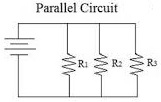# 113th Problem 2016Given the parallel circuit above with the following values:

${ R }_{ 1 }=100\Omega \\ \\ { R }_{ 2 }=300\Omega \\ \\ { R }_{ 3 }=600\Omega \\ \\ { V }_{ T }=150V$

Find the total resistance (${ R }_{ T }$) of the circuit.